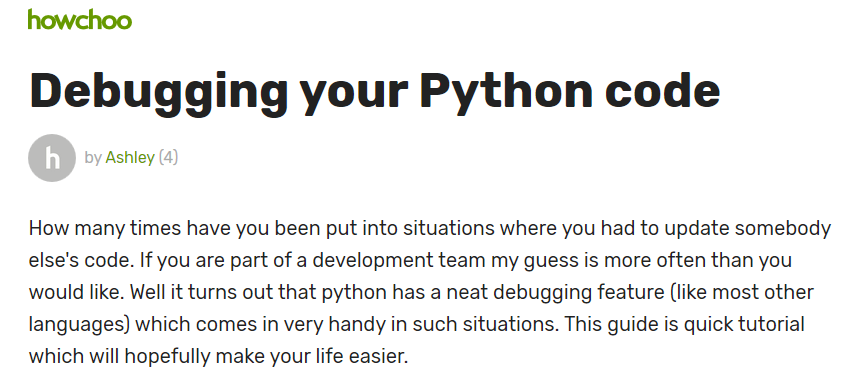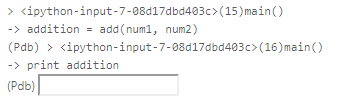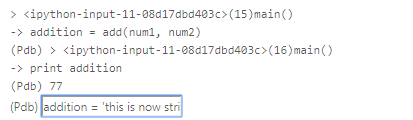# hunkier

Python自带一个调试器, 在Python 3.7之后甚至成为内置调试器. 这就是PDB. 这是使用Python的用户需要掌握的基本技能.In 

``````import pdb

class MyScrapy:
urls = []

def start_url(self, urls):
pdb.set_trace()
for url in urls:
print(url)
self.urls.append(url)

def parse(self):
pdb.set_trace()
for url in self.urls:
result = self.request_something(url)

def request_something(self, url):
print('requesting...')
data = '''<!DOCTYPE html>
<html lang="en">
<meta charset="UTF-8">
<title>Title</title>
<body>
</body>
</html>'''
return data

scrapy= MyScrapy()
scrapy.start_url(["http://www.zone7.cn", "http://www.zone7.cn", "http://www.zone7.cn", "http://www.zone7.cn", ])
scrapy.parse()
``````Python具有一个整洁的调试功能（像大多数其他语言一样），在这种情况下非常方便。本指南是快速教程，希望能让您的生活更轻松

# 1. 一个混乱的程序

（让我们假设用户输入有效值，因此我们不是错误处理）。

In 

``````import sys

return int(num1) + int(num2)

def sub(num1=0, num2=0):
return int(num1) - int(num2)

def main():
#Assuming our inputs are valid numbers
num1 = 0
num2 = 2

subtraction = sub(num1, num2)
print (subtraction)

if __name__ == '__main__':
main()
2
-2
``````

# 2. pdb来了

Python附带了一个名为pdb的有用模块，它基本上是一个交互式源代码调试器。

``````import pdb
pdb.set_trace()
``````

In 

``````import pdb
import sys

return int(num1) + int(num2)

def sub(num1=0, num2=0):
return int(num1) - int(num2)

def main():
#Assuming our inputs are valid numbers
num1 = 33
num2 = 44
pdb.set_trace() # <-- 这个表示添加断点
subtraction = sub(num1, num2)
print (subtraction)

if __name__ == '__main__':
main()
``````# 3. 下一行 - > n# 4. 打印 - > p

``````p num1
``````# 6. 动态分配变量# 8. 其他

• ENTER (重复上次命令)
• c (继续)
• l (查找当前位于哪里)
• s (进入子程序,如果当前有一个函数调用，那么 s 会进入被调用的函数体)
• n(ext) 让程序运行下一行，如果当前语句有一个函数调用，用 n 是不会进入被调用的函数体中的
• r (运行直到子程序结束)
• !<python 命令>
• h (帮助)
• a(rgs) 打印当前函数的参数
• j(ump) 让程序跳转到指定的行数
• l(ist) 可以列出当前将要运行的代码块
• p(rint) 最有用的命令之一，打印某个变量
• q(uit) 退出调试
• r(eturn) 继续执行，直到函数体返回

In 

``````#如发现环境中未安装, 可以运行下方代码来安装ipdb

!pip install ipdb -i https://pypi.tuna.tsinghua.edu.cn/simple
``````